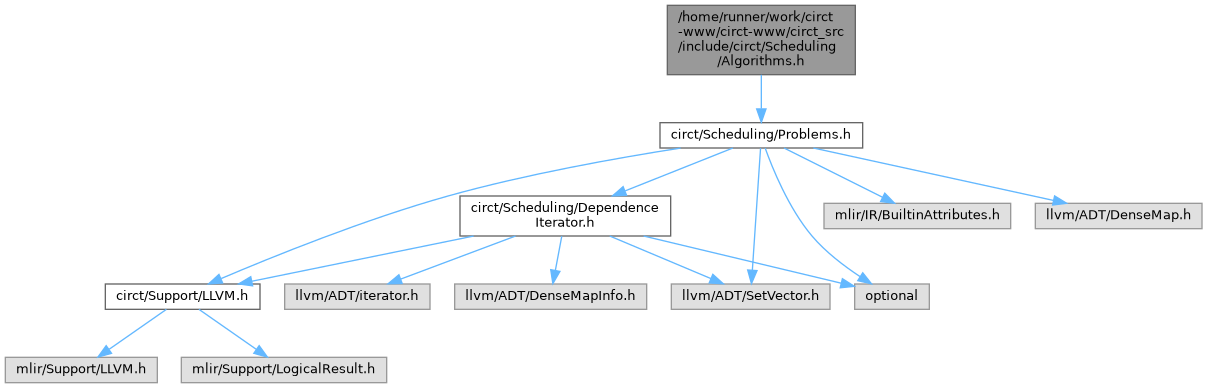CIRCT  18.0.0git
Algorithms.h File Reference
#include "circt/Scheduling/Problems.h"
Include dependency graph for Algorithms.h:This graph shows which files directly or indirectly include this file:Go to the source code of this file.

## Namespaces

circt
This file defines an intermediate representation for circuits acting as an abstraction for constraints defined over an SMT's solver context.

circt::scheduling

## Functions

LogicalResult circt::scheduling::scheduleASAP (Problem &prob)
This is a simple list scheduler for solving the basic scheduling problem. More...

LogicalResult circt::scheduling::scheduleSimplex (Problem &prob, Operation *lastOp)
Solve the basic problem using linear programming and a handwritten implementation of the simplex algorithm. More...

LogicalResult circt::scheduling::scheduleSimplex (CyclicProblem &prob, Operation *lastOp)
Solve the resource-free cyclic problem using linear programming and a handwritten implementation of the simplex algorithm. More...

LogicalResult circt::scheduling::scheduleSimplex (SharedOperatorsProblem &prob, Operation *lastOp)
Solve the acyclic problem with shared operators using a linear programming-based heuristic. More...

LogicalResult circt::scheduling::scheduleSimplex (ModuloProblem &prob, Operation *lastOp)
Solve the modulo scheduling problem using a linear programming-based heuristic. More...

LogicalResult circt::scheduling::scheduleSimplex (ChainingProblem &prob, Operation *lastOp, float cycleTime)
Solve the acyclic, chaining-enabled problem using linear programming and a handwritten implementation of the simplex algorithm. More...

LogicalResult circt::scheduling::scheduleLP (Problem &prob, Operation *lastOp)
Solve the basic problem using linear programming and an external LP solver. More...

LogicalResult circt::scheduling::scheduleLP (CyclicProblem &prob, Operation *lastOp)
Solve the resource-free cyclic problem using integer linear programming and an external ILP solver. More...

LogicalResult circt::scheduling::scheduleCPSAT (SharedOperatorsProblem &prob, Operation *lastOp)
Solve the acyclic problem with shared operators using constraint programming and an external SAT solver. More...